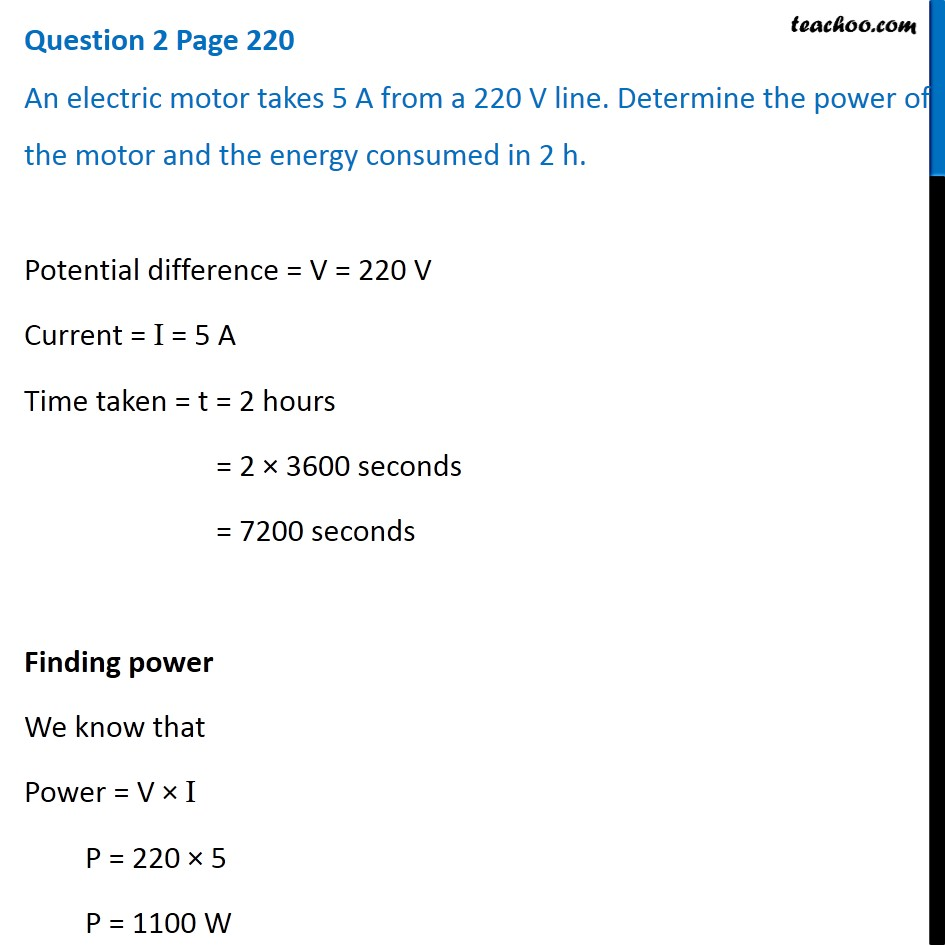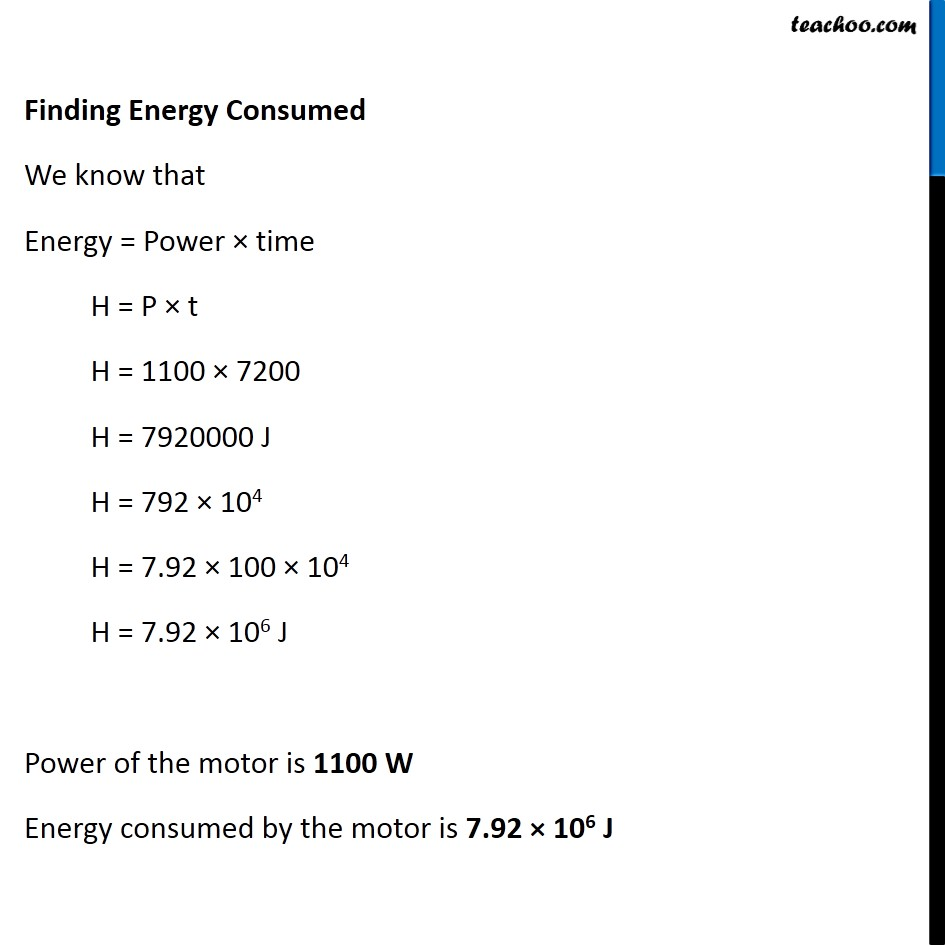Questions from Inside the chapter

Class 10
Chapter 12 Class 10 - Electricity

## An electric motor takes 5 A from a 220 V line. Determine the power of the motor and the energy consumed in 2 hLearn in your speed, with individual attention - Teachoo Maths 1-on-1 Class

### Transcript

Question 2 Page 220 An electric motor takes 5 A from a 220 V line. Determine the power of the motor and the energy consumed in 2 h. Potential difference = V = 220 V Current = I = 5 A Time taken = t = 2 hours = 2 × 3600 seconds = 7200 seconds Finding power We know that Power = V × I P = 220 × 5 P = 1100 W Finding Energy Consumed We know that Energy = Power × time H = P × t H = 1100 × 7200 H = 7920000 J H = 792 × 104 H = 7.92 × 100 × 104 H = 7.92 × 106 J Power of the motor is 1100 W Energy consumed by the motor is 7.92 × 106 J